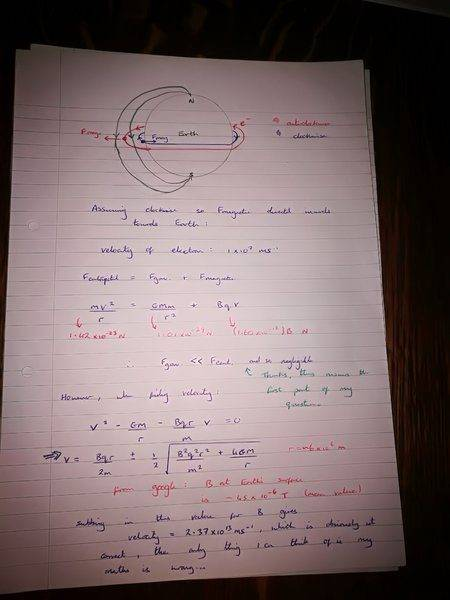# Electrons orbiting the Earth

• Ben King
In summary, the conversation discusses finding the magnetic flux density of the Earth if an electron is orbiting near the surface and how the magnetic force is equal to the centripetal force in the solution. The gravitational force is deemed negligible in this scenario. However, the calculations for finding the velocity of the electron using the same magnetic field strength as the Earth's surface yield an incorrect answer.

#### Ben King

I've been given a question to find the magnetic flux density of the Earth if an electron is orbiting near to the surface. The answer to the question makes the magnetic force equal to the centripetal force and solves for B from there.

However, I am confused to why the gravitational force has no effect and won't the direction of rotation of the electrons have an effect on the direction of the magnetic force and hence the magnitude of the centripetal force? Thanks

The answer given was 8.9e-12 Tesla

Welcome to the PF.

(In the future, please use the Template that you are provided when starting a new schoolwork thread -- it helps to organize your post).

I'd start by comparing the Lorentz force to the gravitational force on the electron. Maybe the gravitational force is negligible...? Can you show us the calculations?

Thanks for the reply. Your suggestion about the gravitational force being negligible is true I think, so that answered that question. However I altered the equation to find velocity of the electron if the magnetic field strength was the same as the surface magnetic field strength of the Earth and got an answer that was way too large, I'll show the equation below. Is there an error in my maths?
##v=(Bqr/2m) \pm 0.5*\sqrt{(Bqr/m)^2+(4Gm/r)} ##
from ##v^2-(Gm/r)-(Bqr/m)v=0##
I googled the mean value for magnetic field strength on the Earth's surface and found it to be ~##45e-6## Tesla, but when I used that number in the formula I got an answer of ##v=2.37e13##, which is obviously not correct. Thanks again